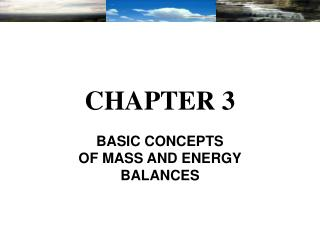DownloadDownload PresentationCHAPTER 3

# CHAPTER 3

Télécharger la présentation## CHAPTER 3

- - - - - - - - - - - - - - - - - - - - - - - - - - - E N D - - - - - - - - - - - - - - - - - - - - - - - - - - -
##### Presentation Transcript

1. CHAPTER 3 BASIC CONCEPTS OF MASS AND ENERGY BALANCES

2. Mass Balance Variations of mass contained whitin the control volume at time “t” Total mass flow entering the system at time “t” Total massflow leaving the system at time “t” = - mcv = mi - me Where : mcv : mass change mi : inputs me: outputs

3. Example : Mass Balance • Mass balance for a system with chemical reaction • A fuel oil is analyzed and is found to contain 87% weight carbon, 11% hydrogen and 1.4% sulfur, with the remainder being non-combustible (inert) material. • The oil is burned with 20% excess air (based on complete combustion of the carbon to CO2, the hydrogen to H2O and the sulphur to SO2). The oil is burned completely, but 5% of the carbon forms CO instead of CO2. • Calculate the molar composition of the exhaust gas leaving the burner.

4. Example : Mass Balance NOTE : INPUT RATE = Qs * Cs + Qw * Cw OUTPUT RATE = Qm * Cm = (Qs + Qw) * Cw DECAY RATE = KCV INPUT RATE = OUTPUT RATE + DECAY RATE

5. Energy Balance for Closed Systems INPUT OUTPUT Netenergy transfered across the system boundary by workdone by the system Netenergy transfered across the system boundary by heattransfer into the system Conservation of energy principle: Time interval variation of the total energy in a system = -

6. Total Energy Variation KE + PE + U = Q – W Where :  KE = Kinetic Energy change  PE = Gravitational Potential Energy change  U = Internal Energy change Q = Heat W = Work All parameter mentioned above, are in Joules, Btu or Calories.

7. Characteristics of Energy Balance Calculations – W is the work transfered from the surroundings to the system. + Q is the heat energy transfered into the system from the surroundings. Therefore : + W is the work done by the system released into the surroundings - Q is the heat energy transfered into the surroundings from the system

8. Example : Energy Balance A mixture of 1 kmol of gaseous methane and 2 kmol of oxygen initially at 25°C and 1 atm burns completely in a closed, rigid container. Heat transfer occurs until the products are cooled to 900K. Determine the amount of heat transfer in kJ. State 1 State 2 Products of combustion T2 P2 1 kmol CH4 (g) 2 kmol O2 T1= 25°C P1= 1 atm

9. Example : Energy Balance Assumptions : • The contents of the closed, rigid container are taken as the system • Kinetic, potential energy effects and work = 0. • Combustion is complete • The reactants and products each form ideal gas mixtures. • The initial and final states are equilibrium states

10. Example : Energy Balance

11. Example : Energy Balance